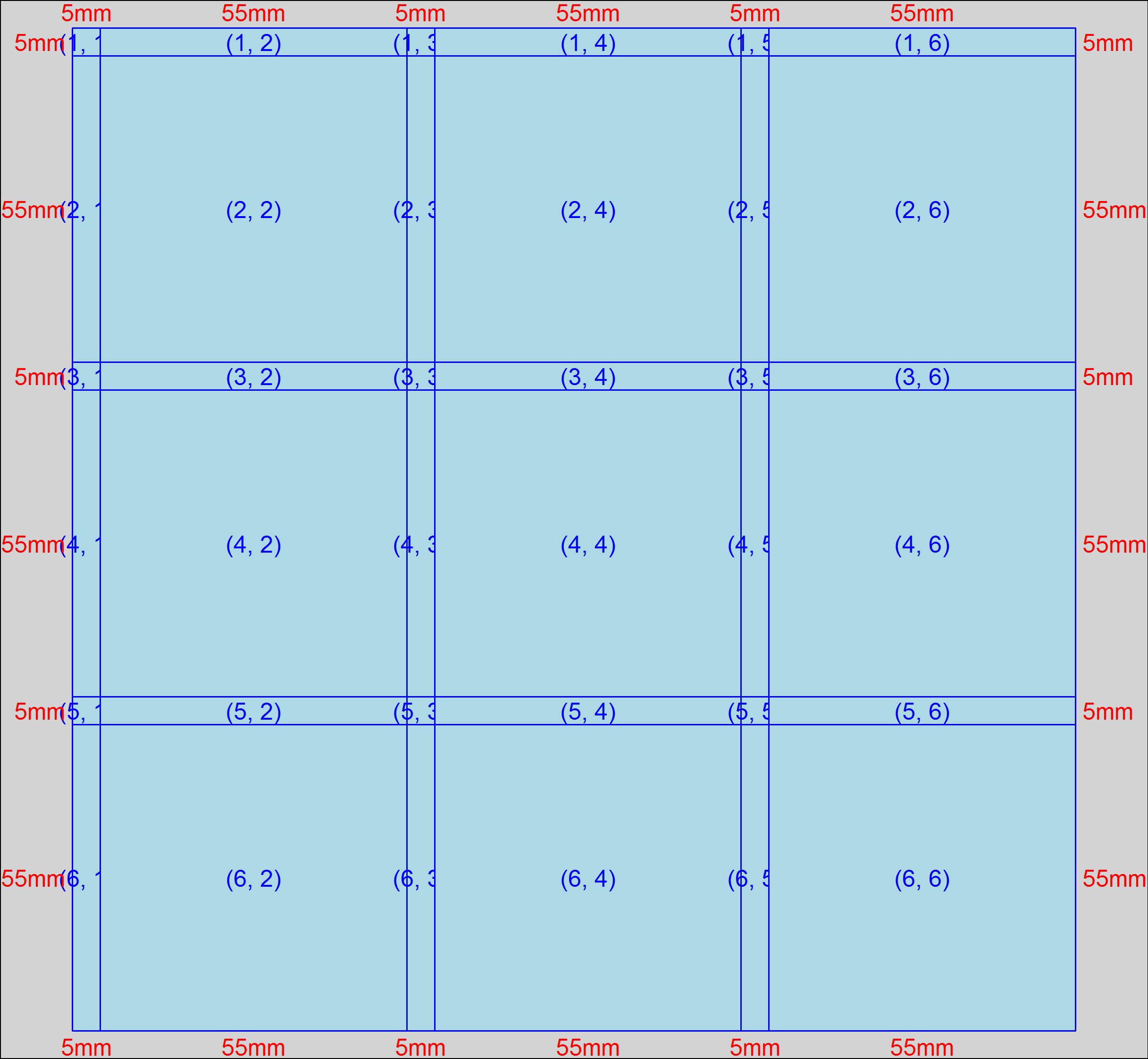# multipanelfigure

Tools to create a layout for figures made of multiple panels, and to fill the panels with base, lattice and ggplot2 plots, grobs, and PNG, JPEG, and TIFF images.

## Installation

To install the package, you first need the devtools package.

``install.packages("devtools")``

Then you can install the multipanelfigure package using

``devtools::install_bitbucket("graumannlabtools/multipanelfigure")``

## Specifying a layout

Layouts are matrices of panels that contains plots, grobs or images. Create them with the `multi_panel_figure` function.

You can specify the total width and height of the figure (the default unit is millimetres):

``````library(multipanelfigure)
figure1 <- multi_panel_figure(
width = 180, height = 180,
columns = 3, rows = 3)``````

Or you can specify widths and heights of individual columns and rows.

``````figure2 <- multi_panel_figure(
width = c(20, 30, 40),
height = c(10, 20, 30))``````

When no panels are filled, printing the figure (by typing its name) displays the layout. Notice that by default a 5 mm spacer is included between rows and between columns. (This can be adjusted using `multi_panel_figure`'s `row_spacing` and `column_spacing` arguments.)

``figure1``An image of the layout of an empty multi-panel figure.

Plots and images are added into the figure by filling panels using `fill_panel`.

lattice plot variables are added directly. The syntax is cleanest using magrittr pipes:

``````library(lattice)
library(magrittr)
a_lattice_plot <- xyplot(height ~ age, Loblolly)
figure1 %<>% fill_panel(a_lattice_plot)``````

ggplot2 plots variables are also added directly. In this case, to put it in the second column from the left (top row), specify the `left_panel` argument.

``````library(ggplot2)
library(magrittr)
a_ggplot <- ggplot(Loblolly, aes(age, height)) + geom_point()
figure1 %<>% fill_panel(a_ggplot, column = 2)``````

Plots created using base graphics must be converted to grid-based plots and captured using `capture_base_plot`.

``````a_base_plot <- capture_base_plot(
with(Loblolly, plot(age, height))
)
figure1 %<>% fill_panel(a_base_plot, column = 3)``````

grid grobs are also added directly. Plots and images can be made to span multiple panels by defining starting and stopping columns using `column` and/or starting and stopping rows using `row`. The following example adds the grob to the second and third rows from the top (first column).

``````library(grid)
a_grob <- linesGrob(arrow = arrow())
figure1 %<>% fill_panel(a_grob, row = 2:3)``````

JPEG, PNG, and TIFF images are added via a string giving their location: either a path to a location on disk, or a URL.

``````figure1 %<>% fill_panel(
``figure1``